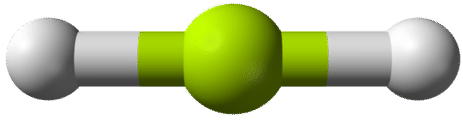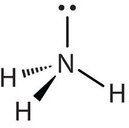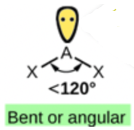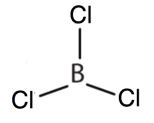# 4.6: Characteristics of Molecules

$$\newcommand{\vecs}{\overset { \rightharpoonup} {\mathbf{#1}} }$$ $$\newcommand{\vecd}{\overset{-\!-\!\rightharpoonup}{\vphantom{a}\smash {#1}}}$$$$\newcommand{\id}{\mathrm{id}}$$ $$\newcommand{\Span}{\mathrm{span}}$$ $$\newcommand{\kernel}{\mathrm{null}\,}$$ $$\newcommand{\range}{\mathrm{range}\,}$$ $$\newcommand{\RealPart}{\mathrm{Re}}$$ $$\newcommand{\ImaginaryPart}{\mathrm{Im}}$$ $$\newcommand{\Argument}{\mathrm{Arg}}$$ $$\newcommand{\norm}{\| #1 \|}$$ $$\newcommand{\inner}{\langle #1, #2 \rangle}$$ $$\newcommand{\Span}{\mathrm{span}}$$ $$\newcommand{\id}{\mathrm{id}}$$ $$\newcommand{\Span}{\mathrm{span}}$$ $$\newcommand{\kernel}{\mathrm{null}\,}$$ $$\newcommand{\range}{\mathrm{range}\,}$$ $$\newcommand{\RealPart}{\mathrm{Re}}$$ $$\newcommand{\ImaginaryPart}{\mathrm{Im}}$$ $$\newcommand{\Argument}{\mathrm{Arg}}$$ $$\newcommand{\norm}{\| #1 \|}$$ $$\newcommand{\inner}{\langle #1, #2 \rangle}$$ $$\newcommand{\Span}{\mathrm{span}}$$$$\newcommand{\AA}{\unicode[.8,0]{x212B}}$$

##### Learning Objectives
• Determine the molecular mass of a molecule.
• Predict the general shape of a simple covalent molecule.
• Predict the polarity of the molecule.
• Compare the properties of ionic and molecular compounds.

Unlike the ions in ionic compounds, which are arranged in lattices called crystals, molecules of covalent compounds exist as discrete units with a characteristic mass and a certain three-dimensional shape.

## Molecular Mass

The mass of a molecule—the molecular mass (sometimes called the molecular weight)—is simply the sum of the masses of its atoms. As with formula masses, it is important that you keep track of the number of atoms of each element in the molecular formula to obtain the correct molecular mass.

##### Example $$\PageIndex{1}$$

What is the molecular mass of each covalent compound?

1. H2O
2. C6H6
3. NO2
4. N2O5
###### Solution

Use the atomic masses from the Periodic Table in Section 2.7.

The molecular formula H2O indicates that there are two hydrogen atoms and one oxygen atom in each molecule. Summing the masses of these atoms,

2 H: 1 O: 2 × 1.01 = 2.02 amu + 16.00 amu 18.02 amu

The molecular mass of H2O is 18.02 amu.

6 C: 6 H: 6 × 12.01 = 72.06 amu 6 × 1.01 = + 6.06 amu 78.12 amu

The molecular mass of C6H6 is 78.12 amu.

1 N: 2 O: 14.01 amu 2 × 16.00 = + 32.00 amu 46.01 amu

The molecular mass of NO2 is 46.01 amu.

2 N: 5 O: 2 × 14.01 = 28.02 amu 5 × 16.00 = + 80.00 amu 108.02 amu

The molecular mass of N2O5 is 108.02 amu.

Note that the two different nitrogen and oxygen compounds in these examples have different molecular masses.

##### Exercise $$\PageIndex{1}$$

What is the molecular mass of each covalent compound?

1. C2H2
2. CO
3. CO2
4. BF3

26.04 amu

28.01 amu

44.01 amu

67.81 amu

## Molecular Shape: VSEPR Theory

Unlike ionic compounds, with their extended crystal lattices, covalent molecules are discrete units with specific three-dimensional shapes. The shape of a molecule is determined by the fact that covalent bonds, which are composed of negatively charged electrons, tend to repel one another. This concept is called the valence shell electron pair repulsion (VSEPR) theory. For example, the two covalent bonds in $$\ce{BeCl2}$$ stay as far from each other as possible, ending up 180° apart from each other. The result is a linear molecule:The three covalent bonds in BF3 repel each other to form 120° angles in a plane, in a shape called trigonal planar:The molecules $$\ce{BeCl2}$$ and $$\ce{BF3}$$ actually violate the octet rule; however, such exceptions are rare and will not be discussed in this text.

Try sticking three toothpicks into a marshmallow or a gumdrop and see if you can find different positions where your “bonds” are farther apart than the planar 120° orientation.

The four covalent bonds in CCl4 arrange themselves three dimensionally, pointing toward the corner of a tetrahedron and making bond angles of 109.5°. CCl4 is said to have a tetrahedral shape:

Table describes how to use the number of atoms around the central atom to determine the geometry of a molecule, as well as examples.
Atoms Around Central Atom Geometry Example
2 $$\ce{AB_2}$$ Linear $$\ce{BeCl_2}$$
3 $$\ce{AB_3}$$ Trigonal Planar $$\ce{BF_3}$$
4 $$\ce{AB_4}$$ Tetrahedral $$\ce{CCl_4}$$

In determining the shapes of molecules, it is useful to first determine the Lewis diagram for a molecule. The shapes of molecules with multiple bonds are determined by treating the multiple bonds as one bond. Thus, formaldehyde (CH2O) has a shape similar to that of BF3. It is trigonal planar.### Molecules With Lone Pairs Around Central Atom

Molecules with lone electron pairs around the central atom have a shape based on the position of the atoms, not the electron pairs. For example, NH3 has one lone electron pair and three bonded electron pairs. These four electron pairs repel each other and adopt a tetrahedral arrangement. However, the shape of the molecule is described in terms of the positions of the atoms, not the lone electron pairs. Thus, NH3 is said to have a trigonal pyramidal shape, not a tetrahedral one.Similarly, H2O has two lone pairs of electrons around the central oxygen atom and two bonded electron pairs. Although the four electron pairs adopt a tetrahedral arrangement, the shape of the molecule is described by the positions of the atoms only. The shape of H2O is bent with an approximate 109.5° angle.In summary, to determine the molecular geometry:

Step 1: Draw the Lewis structure.

Step 2: Count the number of bonds (a double/triple bond counts as one) and lone pairs around the central atom.

Step 3: Use Table 4.5.1 to determine the molecular geometry.If a molecule has 2 bonds and 0 lone pairs, it is linear. If a molecule has 3 bonds and 0 lone pairs, it is trigonal planar. If a molecule has 3 bonds and 1 lone pair, it is bent or angular. If a molecule has 4 bonds and 0 lone pairs, it is tetrahedral. If a molecule has 4 bonds and 1 lone pair, it is trigonal pyramid. If a molecule has 4 bonds and 2 lone pairs, it is bent or angular.

Table 4.5.1: The molecular geometry depends on the number of bonds and lone pairs around the central atom, A.

##### Example $$\PageIndex{1}$$

What is the geometry of the ammonium ion, NH4+? Its Lewis structure is shown below. How is this different from ammonia, NH3?###### Solution

In ammonium ion, the central atom N has 4 bonds and no lone pair. It is equivalent to the below in Table 4.5.1. Hence, this is tetrahedral.In ammonia (NH3), shown below, N has 3 bonds and one lone pair.It is equivalent to the below in Table 4.5.1. Hence, the shape of this molecule is trigonal pyramid.##### Exercise $$\PageIndex{1}$$

What is the molecular shape of nitrosyl chloride, a highly corrosive, reddish-orange gas? Its Lewis structure is shown below.Focus on the central atom, N. It has a double bond to O, count this as one bond. It also has a single bond to Cl. Thus, N has 2 bonds and one lone pair. These 3 electron pairs will spread out 120 degrees from each other. But, since the shape is defined by the arrangement of the atoms only, the shape is bent or angular. If you consult Table 4.5.1, this molecule is equivalent to the below. Hence, two bonds and one lone pair has a bent or angular shape.## Molecular Polarity

In general, a molecule is nonpolar if all its bonds are nonpolar. Examples are I2, O2, H2, CH4, C2H6 and C3H8.

In general, a molecule is polar if it contains polar bonds EXCEPT when the bond polarities cancel each other. As mentioned in Section 4.4, the shape of the CO2 molecule (linear) orients the two C=O polar bonds directly opposite each other, thus cancelling each other's effect. Carbon dioxide (CO2) is a nonpolar molecule.

On the other hand, water (as discussed above) is a bent molecule because of the two lone pairs on the central oxygen atom. Because of the bent shape, the dipoles do not cancel each other out and the water molecule is polar. In the figure below, the individual H-O polar bonds represented by the two red arrows are not directly opposite each other. These two dipoles don't cancel each other out. In fact, the net dipole (blue arrow) points upward. There is a resultant partial positive charge at one end (between the two H atoms) and a partial negative charge on the other end (where O is located). The uneven distribution of charge or the overall dipole is shown by the blue arrow below (Figure 4.5.1). Hence, water is polar (has + and - poles) while carbon dioxide is nonpolar.Figure 4.5.1: The molecular geometry of a molecule (linear vs. bent) affects its polarity.

Similarly, in BF3 (trigonal planar), the effect of a B-F bond is cancelled by the sum of the other two B-F bonds (see video). Hence, a trigonal planar molecule (BF3) is nonpolar because the bond polarities cancel each other, but a trigonal pyramidal molecule (NH3) is polar.Figure 4.5.2: The molecular geometry of a molecule (trigonal planar vs. trigonal pyramid) affects its polarity.

Some other molecules are shown in the figure below. Notice that a tetrahedral molecule such as CCl4 is nonpolar. However, if the peripheral atoms are not of the same electronegativity, the bond polarities don't cancel and the molecule becomes polar, as in CH3Cl.Figure 4.5.3: The same molecular geometry but peripheral bonds are of different electronegativity. CCl4 is nonpolar but CH3Cl is polar.Figure $$\PageIndex{4}$$: Molecules with Polar Bonds. Individual bond dipole moments are indicated in red. Due to their different three-dimensional geometry, some molecules with polar bonds have a net dipole moment (HCl, CH2O, NH3, and CHCl3), indicated in blue, whereas others do not because the bond dipoles cancel due to symmetry (BCl3 and CCl4).

## Physical Properties of Molecular Compounds

The physical state and properties of a particular compound depend in large part on the type of chemical bonding it displays. Molecular compounds, sometimes called covalent compounds, display a wide range of physical properties due to the different types of intermolecular attractions such as different kinds of polar interactions. The melting and boiling points of molecular compounds are generally quite low compared to those of ionic compounds. This is because the energy required to disrupt the intermolecular forces (discussed in Section 8.1) between molecules is far less than the energy required to break the ionic bonds in a crystalline ionic compound. Since molecular compounds are composed of neutral molecules, their electrical conductivity is generally quite poor, whether in the solid or liquid state. Ionic compounds do not conduct electricity in the solid state because of their rigid structure, but conduct well when either molten or dissolved into a solution. The water solubility of molecular compounds is variable and depends primarily on the type of intermolecular forces involved. Substances that exhibit hydrogen bonding or dipole-dipole forces are generally water soluble, whereas those that exhibit only London dispersion forces are generally insoluble. Most, but not all, ionic compounds are quite soluble in water. The table below summarizes some of the differences between ionic and molecular compounds.

Table 4.5.2: Comparison of Ionic and Molecular Compounds
Property Ionic Compounds Molecular Compounds
Type of elements Metal and nonmetal Nonmetals only
Bonding Ionic - transfer of electron(s) between atoms Covalent - sharing of pair(s) of electrons between atoms
Representative unit Formula unit Molecule
Physical state at room temperature Solid Gas, liquid, or solid
Water solubility Usually high Variable
Melting and boiling temperatures Generally high Generally low
Electrical conductivity Good when molten or in solution Poor

In summary, covalent compounds are softer, have lower boiling and melting points, are more flammable, are less soluble in water and do not conduct electricity compared to ionic compounds. The individual melting and boiling points, solubility and other physical properties of molecular compounds depend on molecular polarity.

##### Example $$\PageIndex{2}$$

Describe the shape of each molecule. Is it polar or nonpolar?

1. PCl3
2. CO2
###### Solution
1. The Lewis diagram for PCl3 is as follows:Focus on the central atom, P that has 3 bonds and one lone pair. The four electron pairs arrange themselves tetrahedrally, but the lone electron pair is not considered in describing the molecular shape. Like NH3, this molecule is pyramidal. The 3 P-Cl bonds don't cancel each other. This is polar.

• The Lewis diagram for CO2 is as follows:Focus on the central atom, C. The multiple bonds are treated as one group, hence C has 2 bonds and zero lone pair. CO2 has only two groups of electrons that repel each other. They will direct themselves 180° apart from each other, so CO2 molecules are linear. This is highly symmetrical, with the two opposite dipoles cancelling each other. The CO2 molecule is nonpolar.

##### Exercise $$\PageIndex{2}$$

Describe the shape of each molecule. Is it polar or nonpolar?

1. CBr4
2. BCl3orThe Lewis structure shows 4 groups attached to the central atom, hence tetrahedral. All the 4 groups are identical and the shape is symmetrical. Hence, it is nonpolar.The Lewis diagram shows 3 groups attached to the central atom, hence trigonal planar. All the 3 groups are identical and shape is symmetrical, hence, it is nonpolar.

## Key Takeaways

• A molecule has a certain mass, called the molecular mass.
• Simple molecules have geometries that can be determined from VSEPR theory.
• Polar molecules result from differences in electronegativity of the atoms in the molecule.
• Dipoles that are directly opposite one another cancel each other out.

4.6: Characteristics of Molecules is shared under a CC BY-NC-SA 4.0 license and was authored, remixed, and/or curated by LibreTexts.